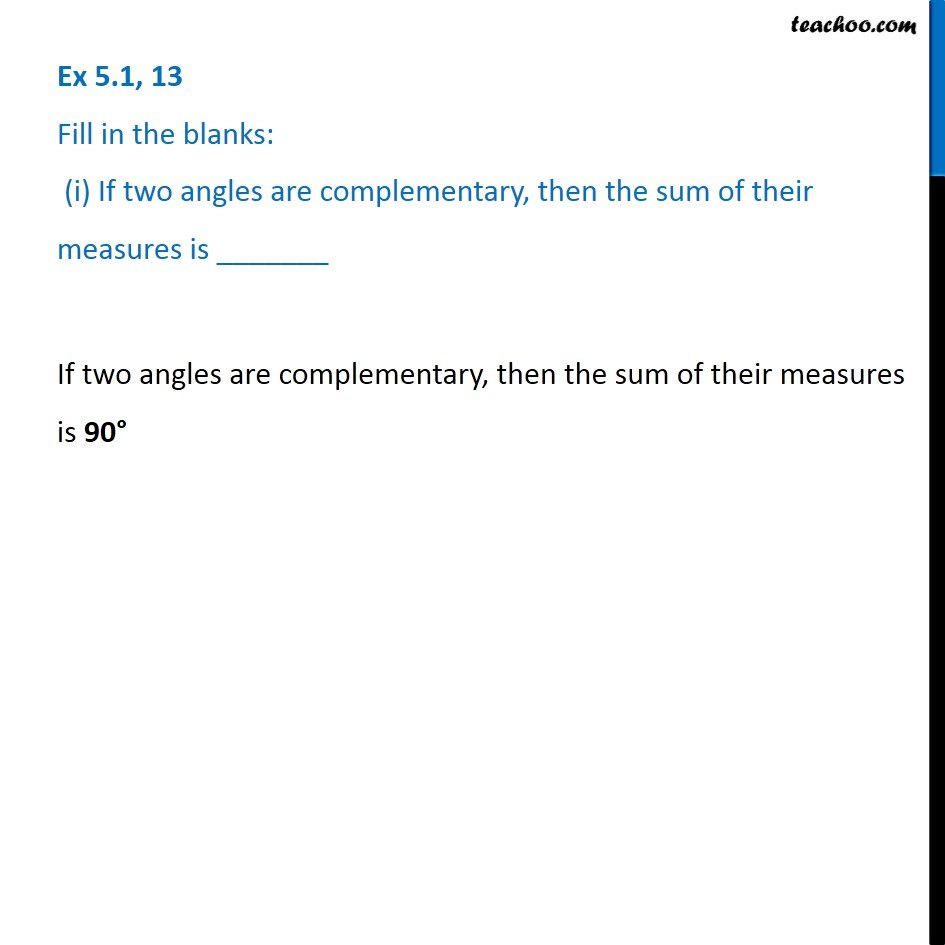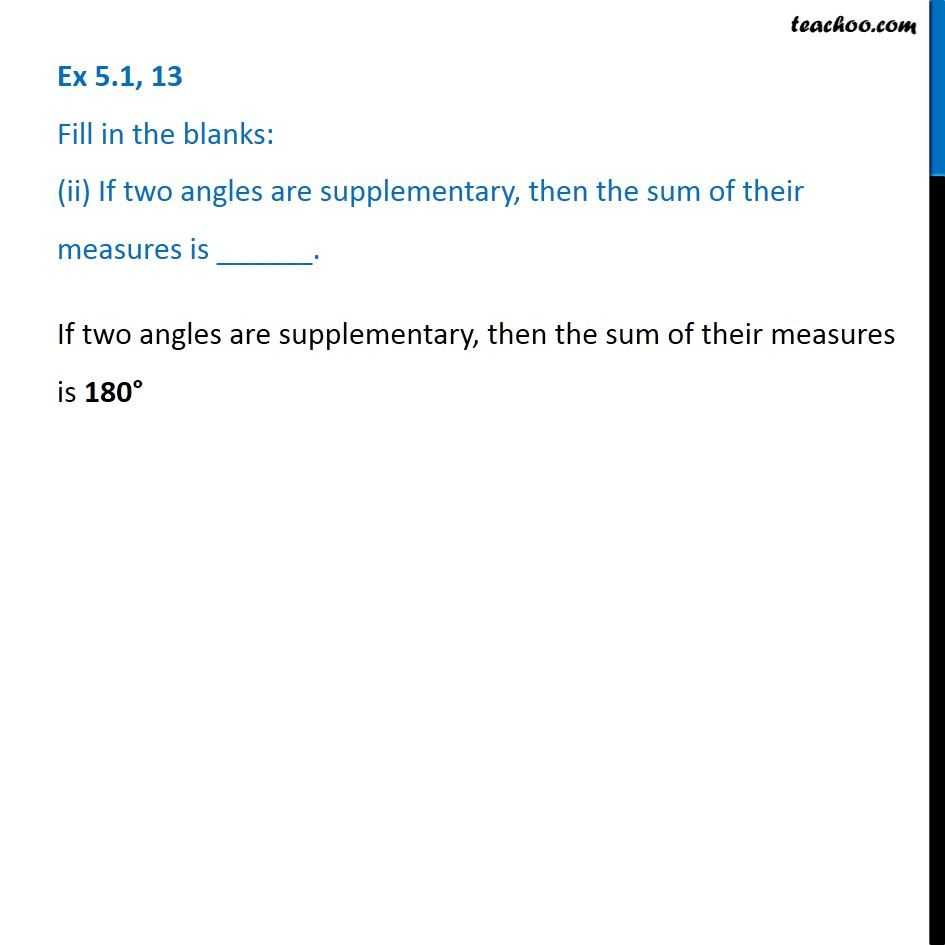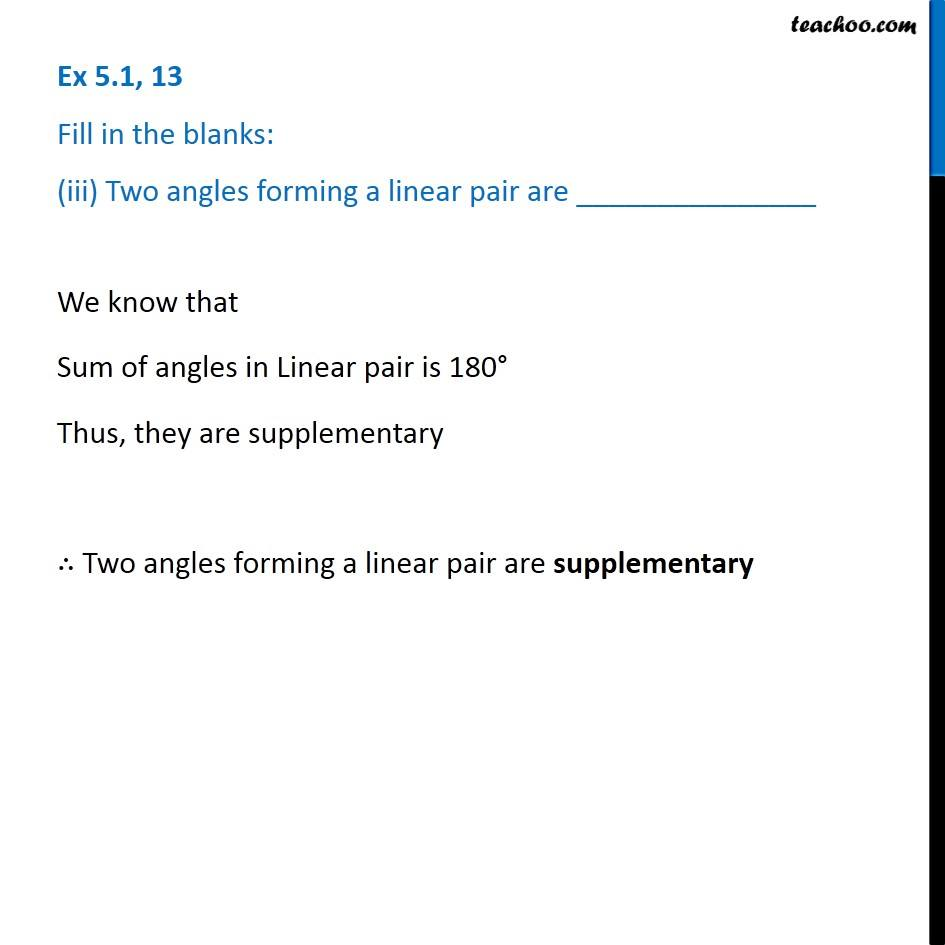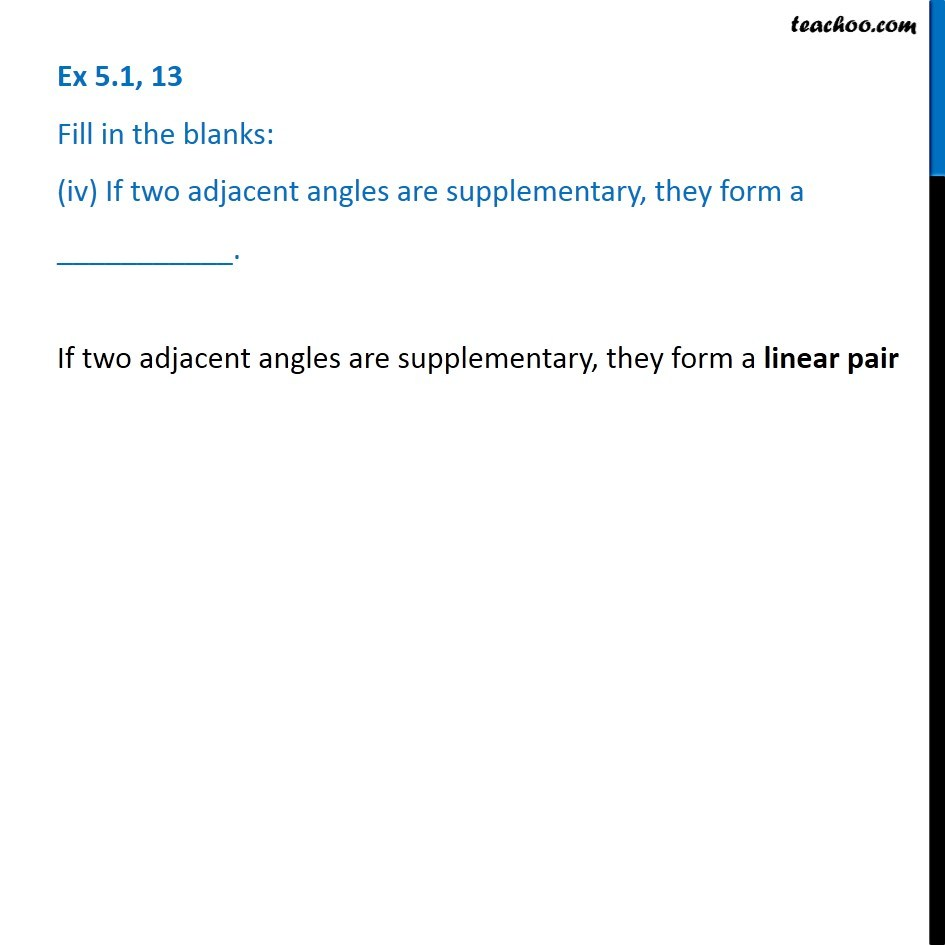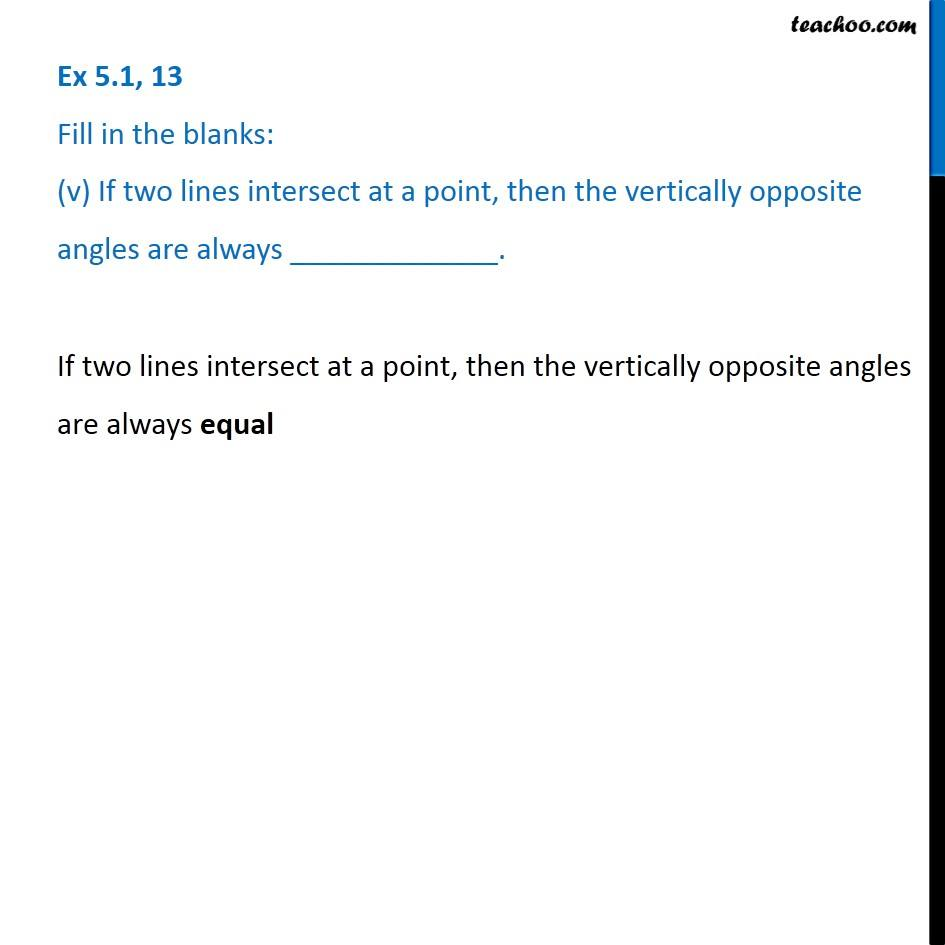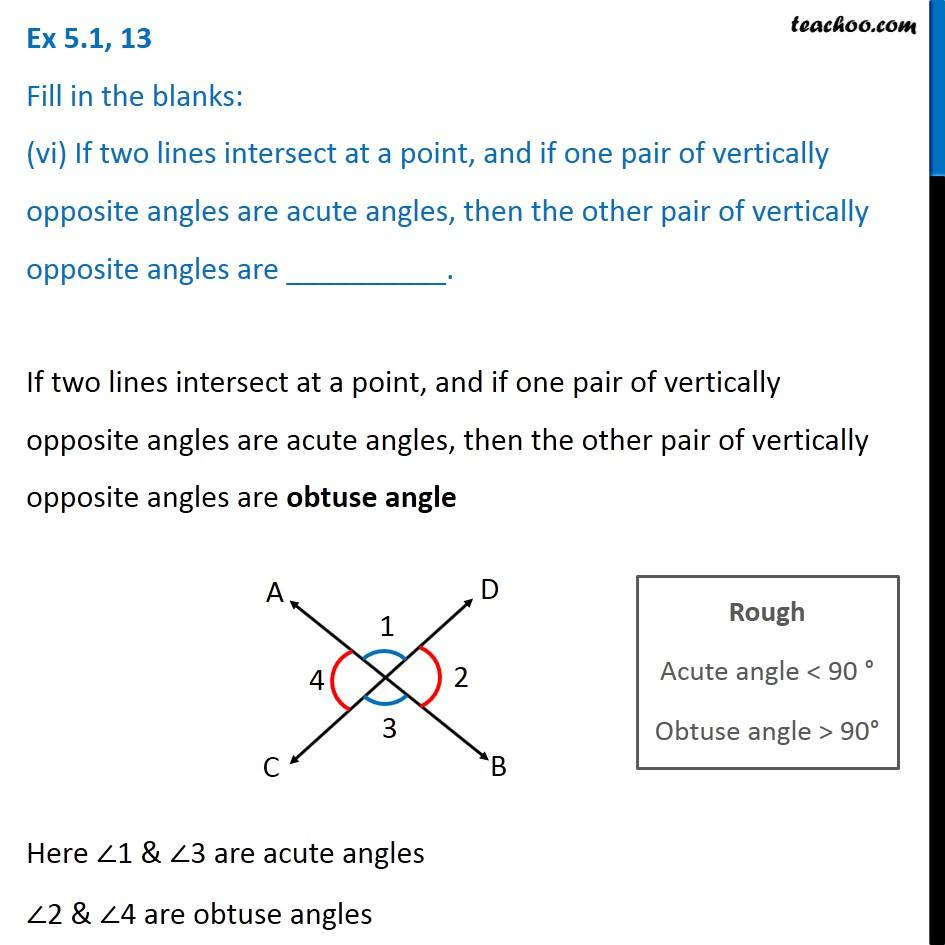Subscribe to our Youtube Channel - https://you.tube/teachoo

1. Chapter 5 Class 7 Lines and Angles
2. Serial order wise
3. Ex 5.1

Transcript

Ex 5.1, 13 Fill in the blanks: (i) If two angles are complementary, then the sum of their measures is _______ If two angles are complementary, then the sum of their measures is 90° Ex 5.1, 13 Fill in the blanks: (ii) If two angles are supplementary, then the sum of their measures is ______. Ex 5.1, 13 Fill in the blanks: (iii) Two angles forming a linear pair are _______________We know that Sum of angles in Linear pair is 180° Thus, they are supplementary ∴ Two angles forming a linear pair are supplementary Ex 5.1, 13 Fill in the blanks: (iv) If two adjacent angles are supplementary, they form a If two adjacent angles are supplementary, they form a linear pair Ex 5.1, 13 Fill in the blanks: (v) If two lines intersect at a point, then the vertically opposite angles are always _____________.If two lines intersect at a point, then the vertically opposite angles are always equal Ex 5.1, 13 Fill in the blanks: (vi) If two lines intersect at a point, and if one pair of vertically opposite angles are acute angles, then the other pair of vertically opposite angles are __________.Ex 5.1, 13 Fill in the blanks: (vi) If two lines intersect at a point, and if one pair of vertically opposite angles are acute angles, then the other pair of vertically opposite angles are __________.Rough Acute angle < 90 ° Obtuse angle > 90°

Ex 5.1